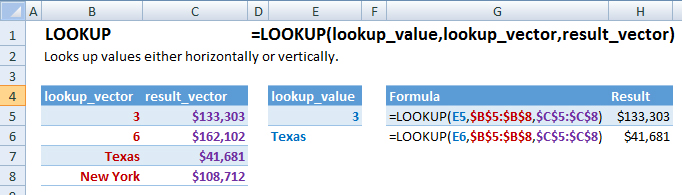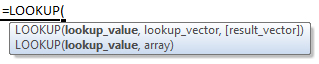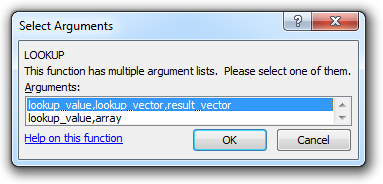# LOOKUP Function Examples – Excel, VBA, & Google Sheets

This Excel Tutorial demonstrates how to use the Excel LOOKUP Function in Excel to look up a value, with formula examples.

## LOOKUP Function Description:

The LOOKUP Function Looks up values either horizontally or vertically.

## Formula Examples:## Syntax and Arguments:

The Syntax for the LOOKUP Formula is:

`=LOOKUP(lookup_value,lookup_vector,result_vector)`

### Function Arguments ( Inputs ):

lookup_value – The value you want to search for.

lookup_vector

result_vector

## LOOKUP Examples in VBA

You can also use the LOOKUP function in VBA. Type:
`application.worksheetfunction.lookup(lookup_value,lookup_vector,result_vector)`
For the function arguments (lookup_value, etc.), you can either enter them directly into the function, or define variables to use instead.

`=AND(`